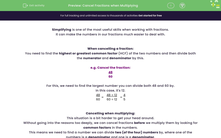# Cancel Fractions when Multiplying

In this worksheet, students will practise cancelling fractions in multiplications sums so that the numbers are lower and, therefore, easier to calculate with.Key stage:  KS 4

Year:  GCSE

GCSE Subjects:   Maths

GCSE Boards:   Pearson Edexcel, OCR, Eduqas, AQA,

Curriculum topic:   Number, Fractions, Decimals and Percentages

Curriculum subtopic:   Structure and Calculation Fractions

Difficulty level:#### Worksheet Overview

Simplifying is one of the most useful skills when working with fractions.

It can make the numbers in our fractions much easier to deal with.

When cancelling a fraction:

You need to find the highest or greatest common factor (HCF) of the two numbers and then divide both the numerator and denominator by this.

e.g. Cancel the fraction:

 48 60

For this, we need to find the largest number you can divide both 48 and 60 by.

In this case, it's 12.

 48 60
=
 48 ÷ 12 60 ÷ 12
=
 4 5

Cancelling when multiplying:

Without going into the reasons too deeply, we can cancel fractions before we multiply them by looking for common factors in the numbers.

This means we need to find a number we can divide two (of the four) numbers by, where one of the numbers is a denominator and one is a denominator.

Huh? Sounds tricky?

Let's look at a few examples to clarify.

e.g. Calculate:

 3 4
x
 5 9

You should notice here that there is 3 on the top of the left-hand fraction and a 9 on the bottom of the right-hand one.

This means we can divide both of these numbers by 3 and we will still get the same answer when we multiply the fractions.

 31 4
x
 5 = 1 x 5 = 5 93 4 3 12

e.g. Calculate:

 1 2
x
 4 5

You should notice here that there is a 2 on the bottom of the left-hand fraction and a 4 on the top of the right-hand one.

This means we can divide both of these by 2 and still get the same answer when we multiply the fractions.

 1 21
x
 42 = 1 x 2 = 2 5 1 5 5

Are these the only scenarios to be aware of?

There could be a combination of both the examples we have shown you.

Don't forget that you could also cancel a fraction if the numerator and the denominator have a common factor within the same fraction.

In this activity, we will practise applying the process shown above to cancel fractions in multiplications sums so that the numbers are lower and, therefore, easier to calculate with.

### What is EdPlace?

We're your National Curriculum aligned online education content provider helping each child succeed in English, maths and science from year 1 to GCSE. With an EdPlace account you’ll be able to track and measure progress, helping each child achieve their best. We build confidence and attainment by personalising each child’s learning at a level that suits them.

Get started